﻿ 改进干扰抵消算法在超低频通信中的应用
 舰船科学技术2020, Vol. 42Issue (2): 155-160PDF

1. 海军工程大学 电子工程学院，湖北 武汉 430033;
2. 中国人民解放军91878部队 广东 湛江 524057

Application of improved interference cancellation algorithm in SLF communication
ZHANG Ning1, JIANG Yu-zhong1, LI Ming-ming2
1. College of Electronic Engineering, Naval University of Engineering, Wuhan 430033, China;
2. No.91878 Unit of the PLA, Zhanjiang 524057, China
Abstract: By analyzing the problem in extracting desired signal in the long-distance marine communication by super-low frequency(SLF) electromagnetic signal, an orthogonal array model of antenna is proposed, and an improved Eigen-subspace Projection(ESP) algorithm is used to process the received signals. The proposed algorithm combines the coefficient constrained adaptive blocking matrix, which can extract the interference information on the main antenna and combines the reference antenna signal to optimize the estimation of the interference subspace. Simulation and experimental results show that the proposed algorithm can effectively cancel wideband interference with smaller angle of the main antenna. In the experiment, when the angle between interference and main antenna is 15°, the SINR is increased by 10.11 dB compared with the traditional algorithm.
Key words: SLF communication     ESP     blocking matrix     antenna array     long-distance marine communication
0 引　言

1 天线与信号模型 1.1 天线建模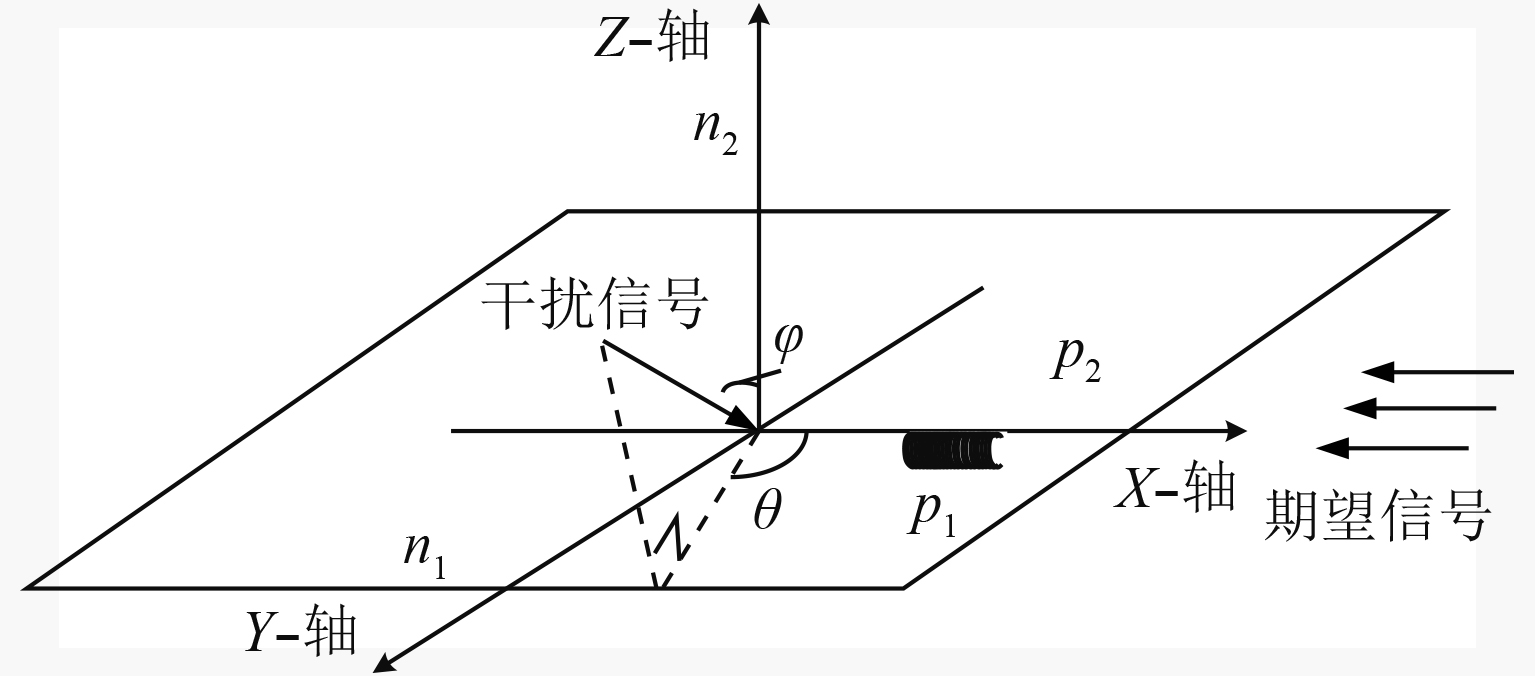图 1 收信天线阵列结构示意图 Fig. 1 Schematic diagram of the receiving antenna′s structure
1.2 信号建模

 $\begin{split} {x_p}\left( n \right) = & s\left( n \right)\; + \;\sum\limits_{j = 1}^K {{P_X}\left( {{\theta _{{w_j}}}} \right){w_j}\left( n \right)} + \sum\limits_{k = 1}^L {P\left( {{\theta _{{r_k}}}} \right){r_k}\left( n \right)} +\\ & {n_\alpha }{\kern 1pt} {\kern 1pt} \left( n \right) + {n_i}\left( n \right) {\text{。}} \end{split}$ (1)

 $\begin{split} & {P_X}\left( {{\theta _{{w_j}}}} \right) = \cos {\theta _{{w_j}}} {\text{，}} \\ & {P_X}\left( {{\theta _{{r_k}}}} \right) = \cos {\theta _{{r_k}}} {\text{。}} \end{split}$ (2)

 $\begin{split} & {x_{ref}}\left( {n - \tau } \right) = \sum\limits_{j = 1}^k {{P_Y}\left( {{\varphi _{{w_j}}},{\theta _{{w_j}}}} \right){w_j}\left( {n - \tau } \right)} + {n_i}\left( {n - \tau } \right) +\\ &\;\sum\limits_{j = 1}^L {{P_Y}\left( {{\varphi _{{r_k}}},{\theta _{{r_k}}}} \right){r_j}\left( {n - \tau } \right)} + {n_\alpha }{\kern 1pt} \left( {n - \tau } \right){\text{。}} \end{split}$ (3)

 $\begin{array}{l} {P_Y}\left( {{\varphi _{{w_j}}},{\theta _{{w_j}}}} \right) = \sin {\theta _{{w_j}}}\sin {\varphi _{{w_j}}} {\text{，}} \\ {P_Y}\left( {{\varphi _{{r_k}}},{\theta _{{r_k}}}} \right) = \sin {\theta _{{r_k}}}\sin {\varphi _{{r_k}}} {\text{。}} \end{array}$ (4)

 ${x_{ref}}\left( {t - \tau } \right) \approx {x_{ref}}\left( t \right){\text{。}}$ (5)
2 干扰抵消算法 2.1 传统的ESP算法

 ${{X}} = {\left[ {{{{x}}_1},{{{x}}_2}, \cdots ,{{{x}}_M}} \right]^{\rm T}} {\text{。}}$ (6)

 ${{{x}}_{ref,i}} = {\left[ {{x_{ref,\;i}}{{\left( t \right)}_{\kern 1pt} },{x_{{\kern 1pt} ref,\;i}}\left( {t - 1} \right), \cdots ,{x_{ref,\;i}}\left( {t - M} \right)} \right]^{\;\rm T}}{\kern 1pt} {\text{。}}$ (7)

 ${\hat R_{ref}} = \;\frac{1}{{{N_{ref}}}}\;\sum\limits_{i{\kern 1pt} = {\kern 1pt} 1}^{{N_{ref}}} {{{{x}}_{ref,\;i}}} {\kern 1pt} {{{x}}_{ref,\;i}}^{\rm H}\;{\text{。}}$ (8)

${{\hat { R}}_{\;ref}}{\kern 1pt}$ 进行特征分解（Eigenvalue decomposition，EVD），得到特征值 $\lambda {{\kern 1pt} _1}{\kern 1pt} ,\;\lambda {{\kern 1pt} _2}{\kern 1pt} ,{\kern 1pt} \; \cdots \;,\;\lambda {{\kern 1pt} _M}{\kern 1pt}$ 以及与特征值相对应的特征向量 ${V_1}{\kern 1pt} ,\;{V_2}{\kern 1pt} ,{\kern 1pt} \; \cdots \;,\;{V_M}$ 。其中会有几个明显较大的特征值对应于空间中的强干扰，取这些特征值所对应的特征向量构成干扰子空间 ${{S}}{\kern 1pt}$ ，余下的 $M - r{\kern 1pt}$ 个特征向量构成信号和噪声子空间 ${{G}}{\kern 1pt}$ 。最后，只要取一路信干噪比最大的主信号投影到信号和噪声子空间 ${{G}}{\kern 1pt}$ 上，即可得到剔除干扰后的序列为：

 ${{{y}}_{out}} = {{{x}}_p} - {{S}}{{{S}}^H}{{{x}}_p}{\rm{ = }}\left( {{{I}} - {\hat { S}}{{{\hat { S}}}^H}} \right){{{x}}_p}{\rm{ = }}{{{P}}_S}{{{x}}_p}{\text{。}}$ (9)

2.2 改进的ESP算法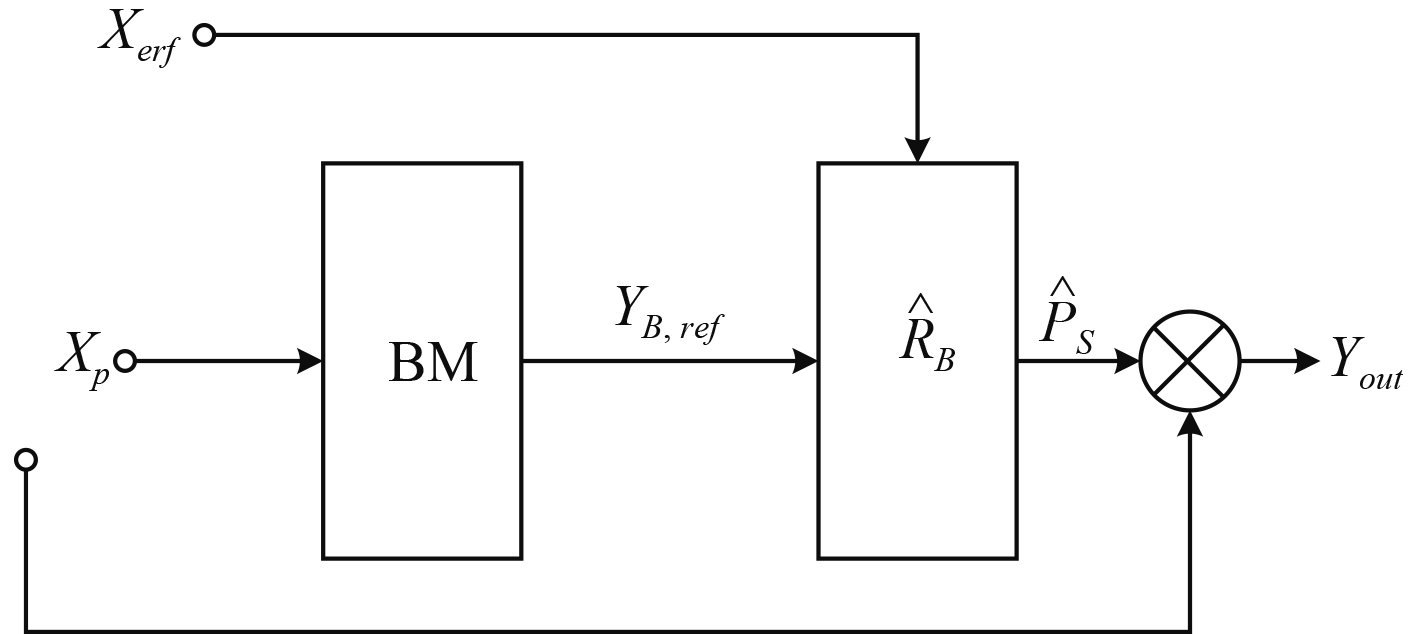图 2 改进的ESP算法原理框图 Fig. 2 Structure of the improved ESP algorithm
2.2.1 系数约束自适应的阻塞矩阵

 ${{b}}_i^{\rm T} \cdot {{1}} = 0{\text{，}}$ (10)

${{b}}_i^{\rm T}$ 之间线性无关时，经过阻塞处理后的信号

 ${{{Y}}_b} = {{{B}}^{\rm T}}{{X}} {\text{，}}$ (11)

 ${{B}} = \left[ {\begin{array}{*{20}{c}} 1&{ - 1}&0& \cdots &0 \\ 0&1&{ - 1}& \ddots &0 \\ \vdots & \ddots & \ddots & \ddots & \vdots \\ 0& \cdots &0&1&{ - 1} \end{array}} \right] {\text{，}}$ (12)

 ${{{B}}_M} = \left[ {\begin{array}{*{20}{c}} 1&{{\alpha _1}}&0& \cdots &0 \\ 0&1&{{\alpha _2}}& \ddots &0 \\ \vdots & \ddots & \ddots & \ddots & \vdots \\ 0& \cdots &0&1&{{\alpha _{K - 1}}} \end{array}} \right] {\text{。}}$ (13)

${{{b}}_i}$ 代表阻塞矩阵 ${B_M}$ 的第i行，则对于 $\alpha$ 的问题求解被描述如下：

 $\left\{ \begin{array}{l} \min {\kern 1pt} {\kern 1pt} {\kern 1pt} {\kern 1pt} {\kern 1pt} {\kern 1pt} SIN{R_F}\left( {{{{b}}_i}{{{X}}_p},f} \right) {\text{，}} \\ {\rm s.t.}{\kern 1pt} {\kern 1pt} {\kern 1pt} {\kern 1pt} {\kern 1pt} {\kern 1pt} {\xi _d} < {\alpha _i} < {\xi _t} {\text{。}} \end{array} \right.$ (14)

 $SIN{R_F}\left( {{{x}},f} \right) = 10\lg \frac{{{P_s}}}{{{P_n}}}{\kern 1pt} {\kern 1pt} {\kern 1pt} \left( {\rm dB} \right) {\text{。}}$ (15)

 ${{{Y}}_{B,ref}} = {{{B}}_M}^{\rm T}{{{X}}_p} {\text{，}}$ (16)

2.2.2 联合优化估计干扰子空间

 ${{\hat { R'}}_{ref}} = \frac{1}{{M - K + 1}}\left( {\sum\limits_{i = 1}^{{N_{ref}}} {{{{x}}_{ref,\;i}}} {\kern 1pt} {{{x}}_{ref,\;i}}^H + {{{Y}}_{B,ref}}{{{Y}}_{B,ref}}^{\rm H}} \right){\text{。}}$ (17)

${{\hat { R'}}_{ref}}{\kern 1pt}$ 是优化估计后的协方差矩阵。按照ESP算法，进行EVD即可求得新的干扰子空间。

3 仿真及实测结果验证

3.1 仿真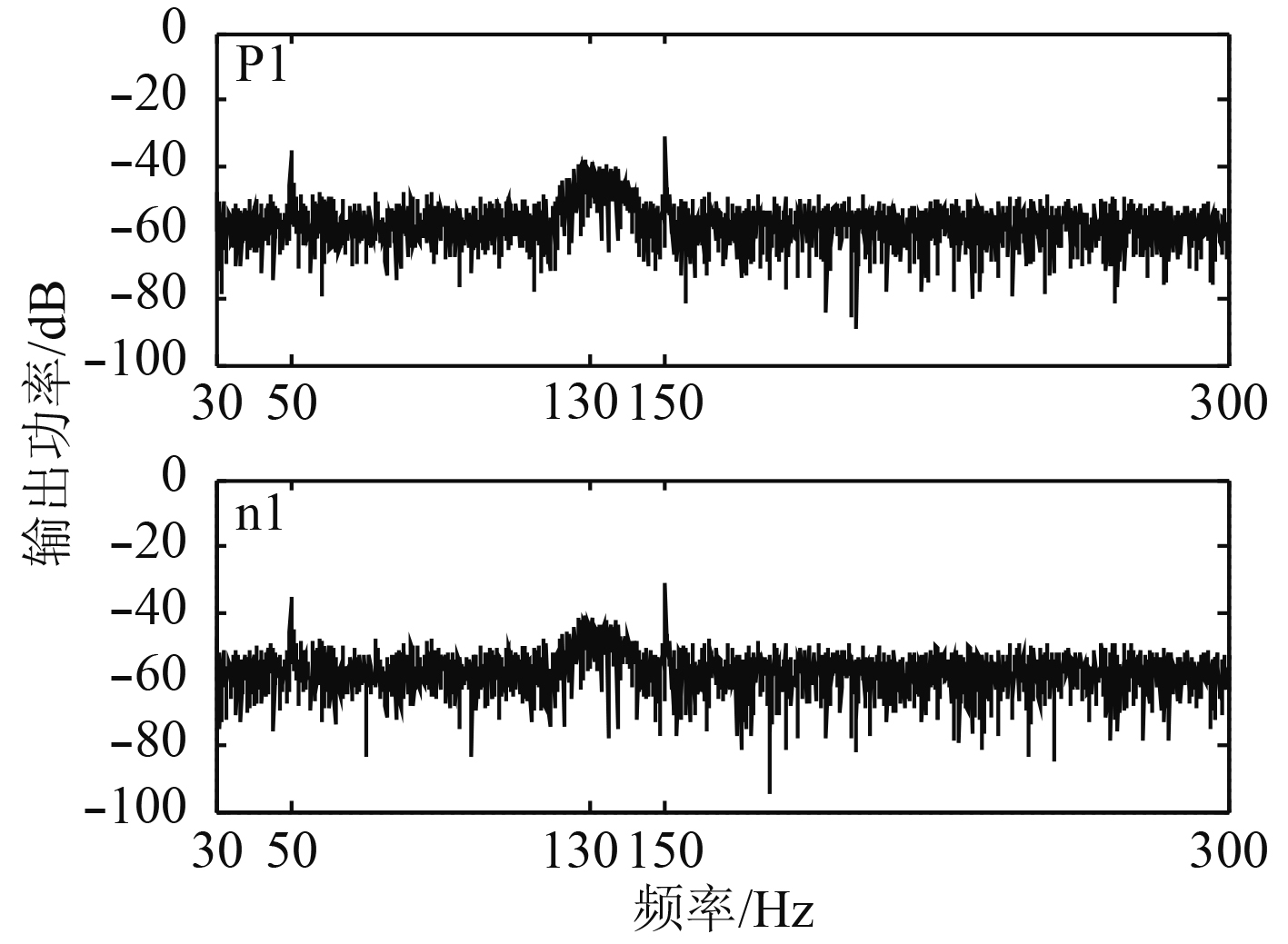图 3 主天线和参考天线的仿真信号模型 Fig. 3 The model of simulation signal of main and reference antenna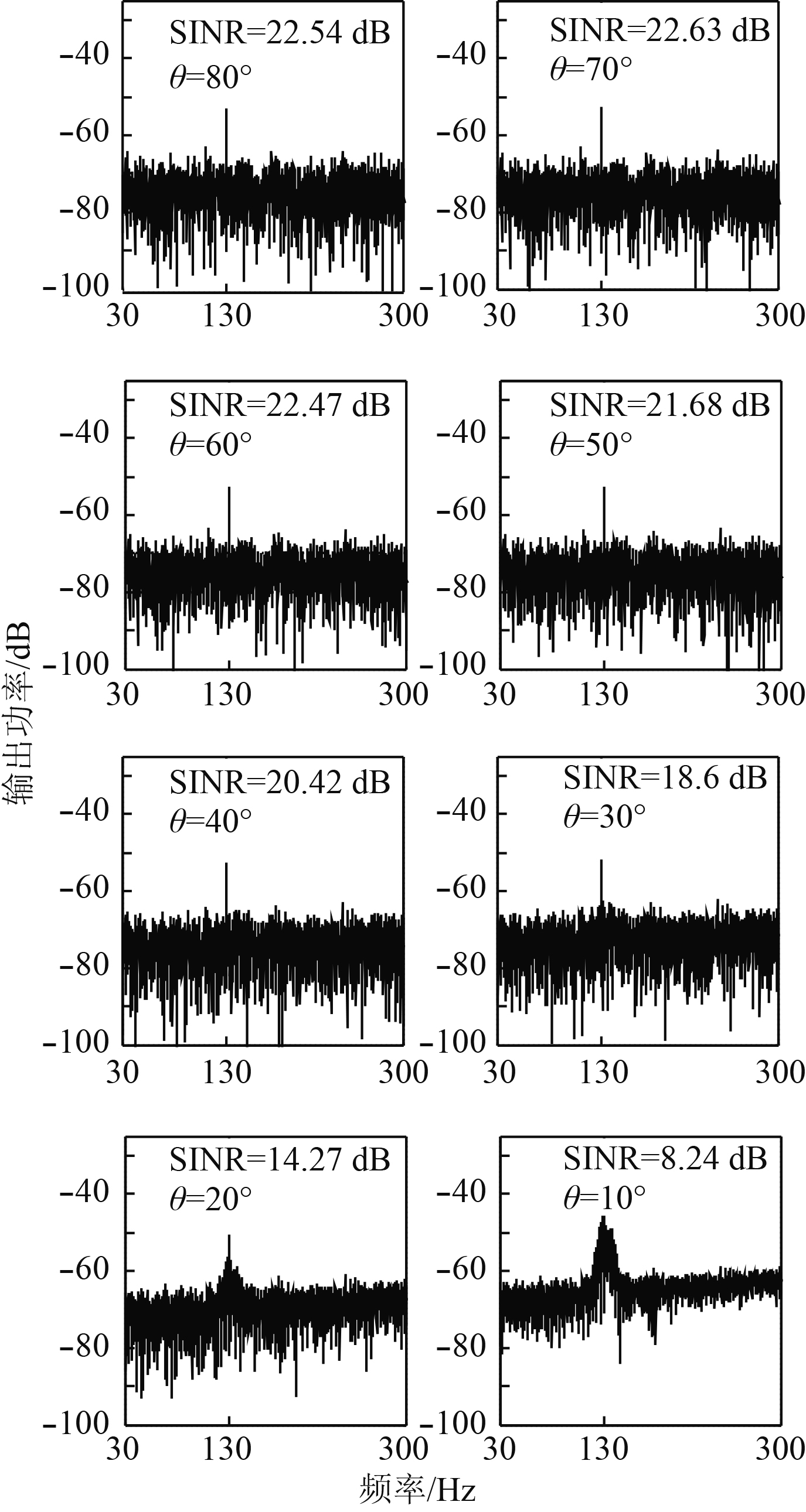图 4 传统ESP算法处理不同入射角度的干扰的性能表现 Fig. 4 Performance of traditional ESP algorithm in dealing with interference at different angles of incidence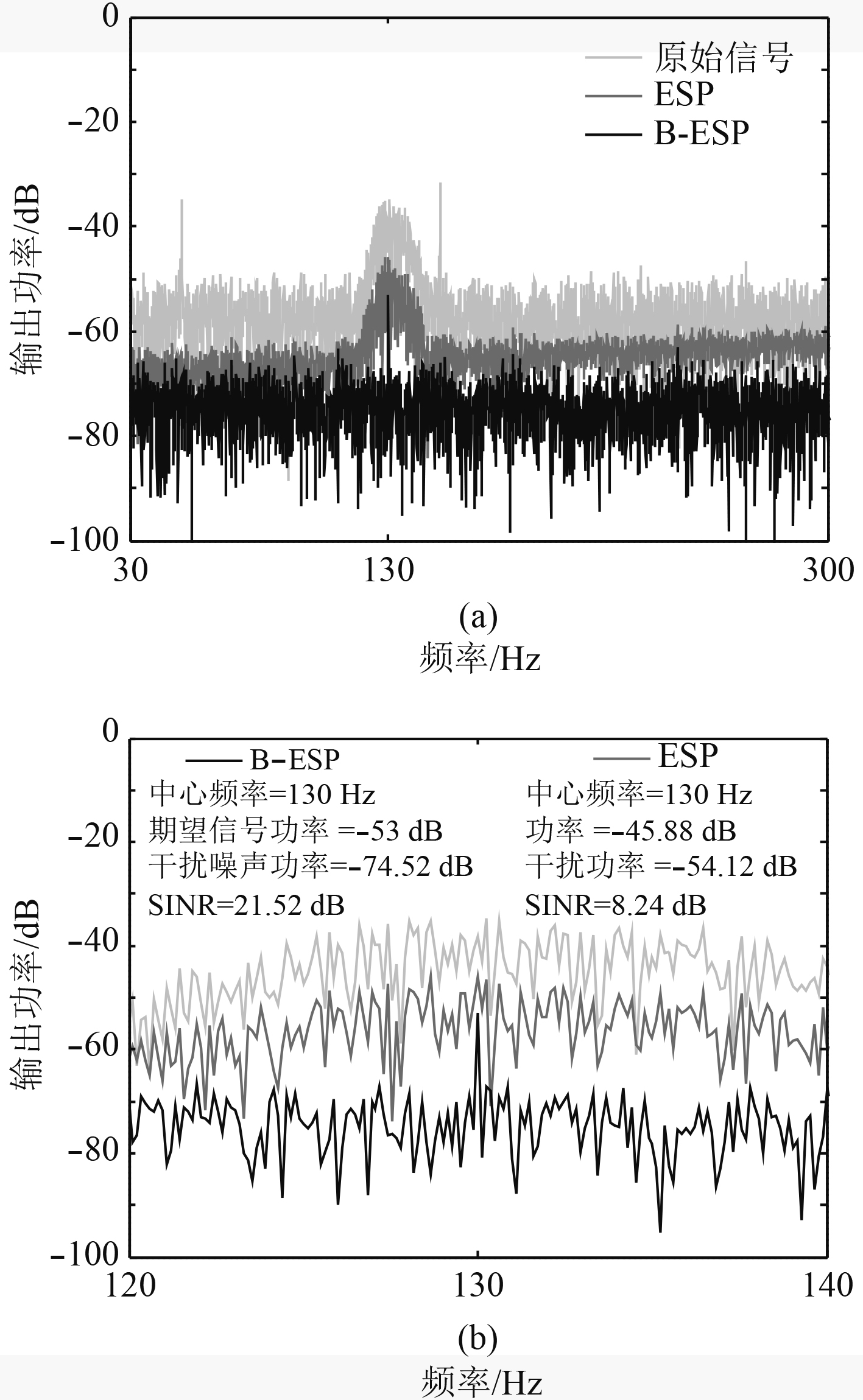图 5 两种算法的干扰抵消效果对比（ $\theta = 10^\circ {\kern 1pt}$ ） Fig. 5 Comparison of the performance of the two algorithms

3.2 实验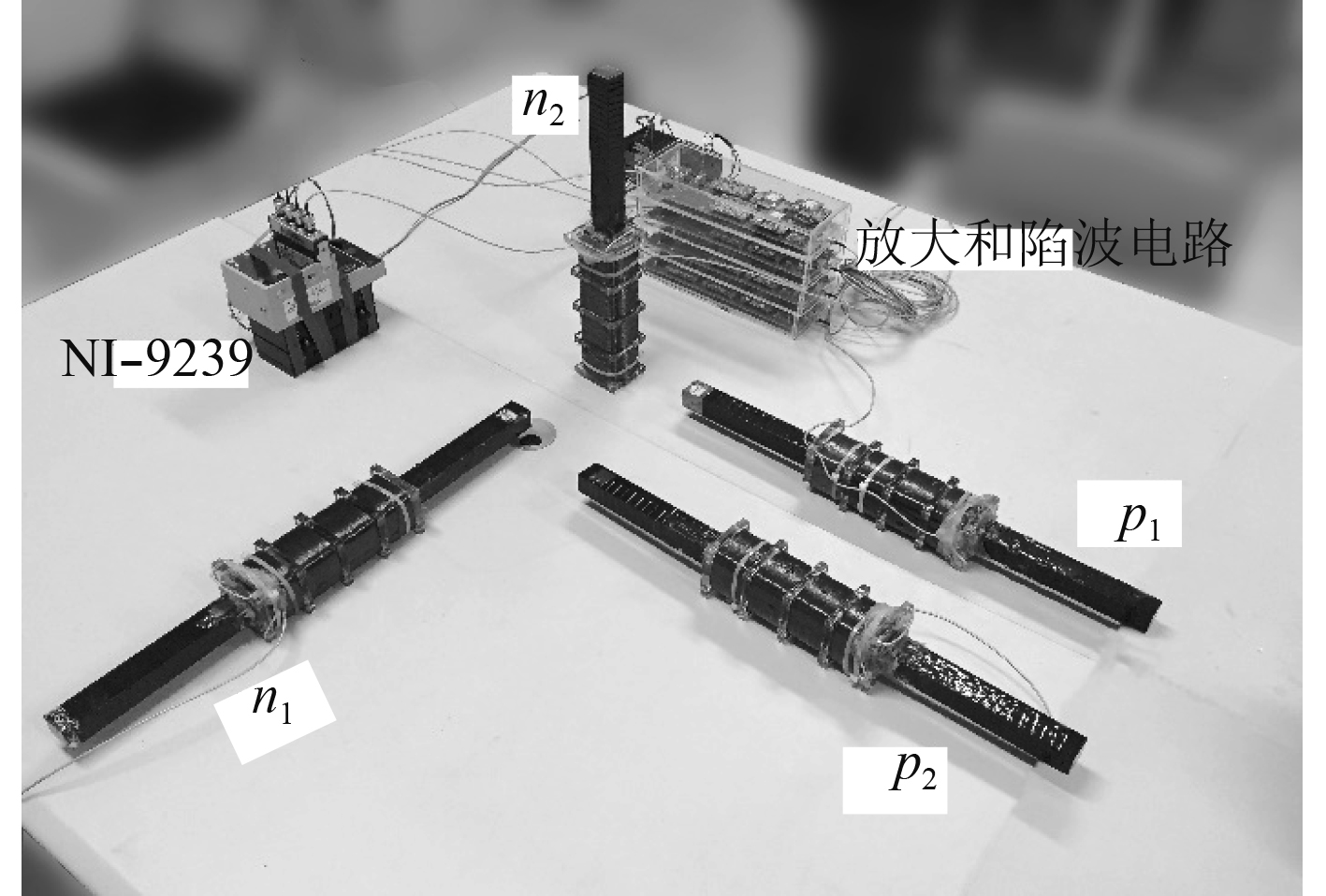图 6 接收天线阵列 Fig. 6 Receiving antenna arrays表 1 B-ESP和ESP算法的性能对比 Tab.1 Comparison of the performance of the B-ESP and ESP algorithms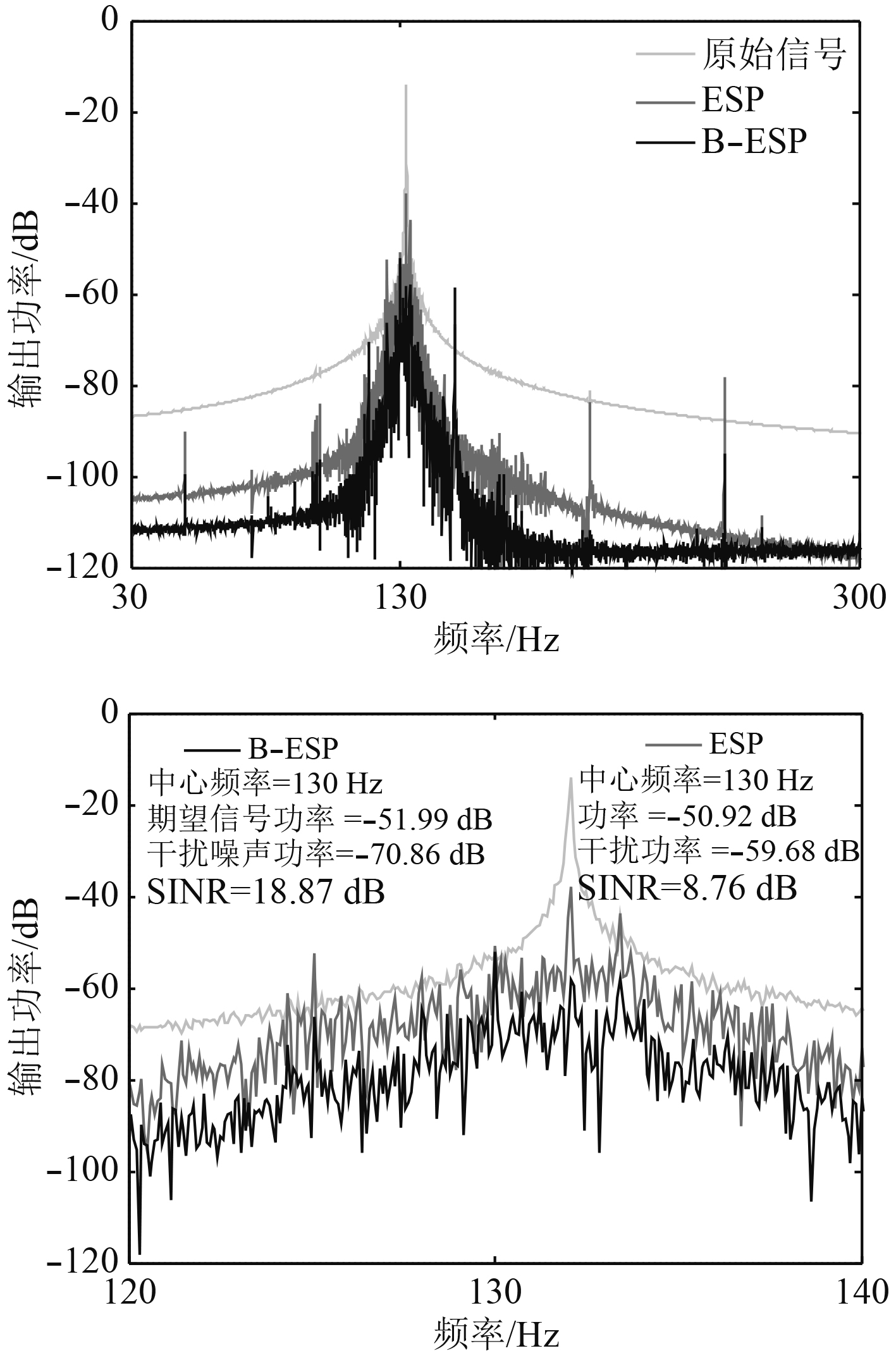图 7 $\theta = 15^\circ {\kern 1pt}$ 实验条件下2种算法的干扰抵消效果对比 Fig. 7 Comparison of the performance of two algorithms in experiment
4 结　语

  高化猛, 李智. 一种Ka频段海上卫星通信抗雨衰编码方案[J]. 舰船科学技术, 2011, 33(12): 76-78. GAO Hua-meng, LI Zhi. An anti-rain attenuation encoding solution for sea Ka-band satellite communication[J]. Ship Science and Technology, 2011, 33(12): 76-78. DOI:10.3404/j.issn.1672-7649.2011.12.017  YAN L, WAYNERT J, SUNDERMAN C, et al. Statistical analysis and modeling of VLF/ELF noise in coal mines for through-the-earth wireless communications[C]// Industry Applications Society Meeting. IEEE, 2014: 1-5.  房贻广, 颜普, 刘武, 等. 基于平稳小波变换和亮度序的局部特征描述子[J]. 计算机工程, 2017, 43(11): 272-276. FANG Yiguang, YAN Pu, LIU Wu, et al. Local feature descriptor based on stationary wavelet transform and intensity orde[J]. Computer Engineering, 2017, 43(11): 272-276. DOI:10.3969/j.issn.1000-3428.2017.11.043  SINGH O, SUNKARIA R K. Powerline interference reduction in ECG signals using empirical wavelet transform and adaptive filtering[J]. Journal of Medical Engineering & Technology, 2015, 39(1): 60-68.  FRIDMAN P A, BAAN W A. RFI mitigation methods in radio astronomy[J]. Journal of Astronomy & Astrophysics, 2001, 378(1): 327-344.  QIAN J, HE Z. Mainlobe interference suppression with eigenprojection algorithm and similarity constraints[J]. Electronics Letters, 2016, 52(3): 228-230. DOI:10.1049/el.2015.2951  周春晖, 李飞, 李宁, 等. 改进的基于特征子空间的SAR图像射频干扰抑制算法[J]. 雷达学报, 2018, 7(2): 235-243. ZHOU C, LI F, LI N, et al. Modified eigensubspace-based approach for radio-frequency interference suppression of sar image[J]. Journal of Applied Remote Sensing, 2018, 7(2): 235-243.  ZHOU C, LI F, LI N, et al. Improved eigensubspace-based approach for radio frequency interference filtering of synthetic aperture radar images[J]. Journal of Applied Remote Sensing, 2017, 11(2): 025004. DOI:10.1117/1.JRS.11.025004  HOSHUYAMA O, SUGIYAMA A. A robust adaptive beamformer for microphone arrays with a blocking matrix using constrained adaptive filters[J]. IEEE Trans Signal Process, 1999, 47(10): 2677-2684. DOI:10.1109/78.790650  YENCHEK M R, HOMCE G T, DAMIANO N W, et al. NIOSH-Sponsored Research in Through-the-Earth Communications for Mines: A Status Report[J]. IEEE Transactions on Industry Applications, 2012, 48(5): 1700-1707. DOI:10.1109/TIA.2012.2209853  袁翊. 超低频和极低频电磁波的传播及噪声[M]. 北京: 国防工业出版社, 2011.